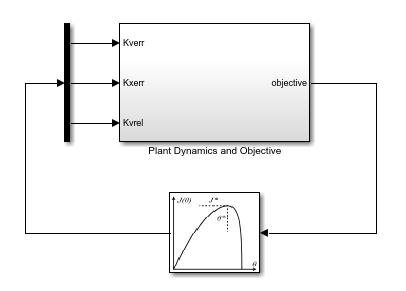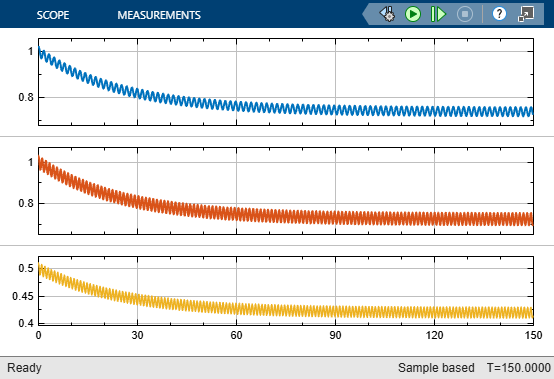# Adaptive Cruise Control Using Extremum Seeking Control

This example shows how to implement adaptive cruise control using an extremum seeking control (ESC) approach. In this example, the goal is to make an ego car travel at a set velocity while maintaining a safe distance from a lead car by controlling longitudinal acceleration and braking.

Adaptive cruise control (ACC) is a system designed to help vehicles maintain a safe following distance and stay within the speed limit. A vehicle equipped with an ACC system (ego car) uses radar to measure relative distance (${D}_{rel}$) and relative velocity (${V}_{rel}$) with respect to the leading vehicle. The ACC system is designed to maintain a desired cruising speed (${V}_{set}$) or maintain a relative safe distance (${D}_{safe}$) from the leading car. The switch in the control objective is determined based on the following conditions.

• If ${D}_{rel}>{D}_{safe}$, the ACC system follows the desired reference cruise velocity commanded by the driver.

• If ${D}_{rel}<{D}_{safe}$, the ACC system controls the relative position of the ego car with respect to the lead car.

This example uses the same ego and lead car model as the Adaptive Cruise Control System Using Model Predictive Control (Model Predictive Control Toolbox).

Implement the longitudinal vehicle dynamics as a simple second-order linear model.

`G = tf(1,[0.5,1,0]);`

Configure the ACC parameters for the example.

```D_default = 10; % Default spacing (m) t_gap = 1.4; % Time gap (s) v_set = 30; % Driver-set velocity (m/s) amin_ego = -3; % Minimum acceleration for driver comfort (m/s^2) amax_ego = 2; % Maximum acceleration for driver comfort (m/s^2) Ts = 0.1; % Sample time (s) Tf = 150; % Duration (s) ```

Specify the initial position and velocity for both the lead car and ego car.

```x0_lead = 50; % Initial lead car position (m) v0_lead = 25; % Initial lead car velocity (m/s) x0_ego = 10; % Initial ego car position (m) v0_ego = 20; % Initial ego car velocity (m/s)```

### ACC Using Extremum Seeking Control

An extremum seeking controller achieves satisfactory control performance by adjusting control parameters to maximize an objective function in real time. For this example, use the following objective function, which depends on relative distance, safe distance, relative velocity, and set velocity.

`$J=-\int {Q}_{d}{\left({D}_{rel}-{D}_{safe}\right)}^{2}+{Q}_{v}{\left({v}_{rel}-{v}_{set}\right)}^{2}$`

Here, ${\mathit{Q}}_{\mathit{d}}$ and ${\mathit{Q}}_{\mathit{v}}$ are objective function weights for the distance error and velocity error terms, respectively.

```Qd = 0.5; Qv = 1;```

The extremum seeking controller adapts the following controller gains.

• ${K}_{xerr}$ — Position error gain

• ${K}_{verr}$ — Velocity error gain

• ${K}_{vrel}$ — Relative velocity gain

Specify initial guesses for the gain values.

```Kverr = 1; % ACC velocity error gain Kxerr = 1; % ACC spacing error gain Kvrel = 0.5; % ACC relative velocity gain```

### Specify Extremum Seeking Control Parameters

Simulink Control Design software implements the ESC algorithm using the Extremum Seeking Control block. Configure the parameters for this block.

Specify the number of parameters to tune (the three controller gains). The controller uses a separate tuning loop for each parameter.

`N = 3;`

Specify the initial conditions for the parameter update integrators by scaling the initial gain values with respect to the learning rate for each parameter `lr`.

```lr = 0.02*[2 3 1]; IC = [Kverr,Kxerr,Kvrel];```

Configure the demodulation and modulation signals by specifying their frequencies (`omega`), phases (`phi_1` and `phi_2`), and amplitudes (`a` and `b`). Each parameter must use a different forcing frequency. For this example, use the same modulation and demodulation phases and amplitudes for all parameters.

```omega = 0.8*[5,7,8]; % Forcing frequency (rad/s) a = 0.01; % Demodulation amplitude b = 0.5*lr; % Modulation amplitude phi_1 = 0; % Demodulation phase (rad) phi_2 = pi/4; % Modulation phase (rad)```

Use a low-pass filter to remove high-frequency noise from the demodulated signal and a high-pass filter to remove bias from the perturbed objective function signal. Specify the cutoff frequencies for these filters.

```omega_lpf = 0.04; omega_hpf = 0.01;```

### Simulate Adaptive Cruise Control System

To simulate the ESC adaptive cruise controller, open the `ExtremumSeekingControlACC` model.

```mdl = 'ExtremumSeekingControlACC'; open_system(mdl)```The Plant Dynamics and Objective subsystem contains the ACC models and computes the objective function for the ESC algorithm.

`open_system([mdl '/Plant Dynamics and Objective'])`Simulate the model. During the simulation, the lead car velocity varies sinusoidally. Therefore, the ego car must adjust its velocity to compensate.

`sim(mdl);`

The following plot shows the relative distance between the lead and ego cars and the safe distance.

• The safe distance varies as the ego car velocity changes.

• The relative distance between the ego and lead cars occasionally drops slightly below the safe distance. This result is because the ACC system enforces the relative distance using a soft constraint.

`open_system([mdl '/Plant Dynamics and Objective/Simulation results/Distance'])`View the velocities of the ego and lead cars along with the ego car set velocity. To maintain a safe distance the ACC system adjusts the ego car velocity as the lead car velocity changes. When the lead car velocity is greater than the set velocity, the ego car stops tracking the lead car velocity and cruises at the set velocity.

`open_system([mdl '/Plant Dynamics and Objective/Simulation results/Velocity'])`The next plot shows the cost function that ESC seeks to optimize when searching for optimal control gains.

`open_system([mdl '/Plant Dynamics and Objective/Simulation results/Cost'])`View the resulting controller gains, which adapt over the course of the simulation. The top plot is ${K}_{verr}$, the middle plot is ${K}_{xerr}$, and the bottom plot is ${K}_{vrel}$. Fluctuations in the gain values are due to the modulation signals from the Extremum Seeking Control block.

`open_system([mdl '/Plant Dynamics and Objective/Gains'])``bdclose('ExtremumSeekingControlACC')`